# GMAT Math : DSQ: Understanding factoring

## Example Questions

### Example Question #1 : Dsq: Understanding Factoring

Ifandare positive, what is the value of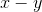?

(1)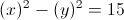(2)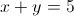A. Statement (1) ALONE is sufficient, but statement (2) alone is not sufficient.

B. Statement (2) ALONE is sufficient, but statement (1) alone is not sufficient.

C. BOTH statements TOGETHER are sufficient, but NEITHER statement ALONE is sufficient.

D. EACH statement ALONE is sufficient.

E. Statements (1) and (2) TOGETHER are NOT sufficient.

C. BOTH statements TOGETHER are sufficient, but NEITHER statement ALONE is sufficient.

Explanation:

For statement (1), we can factor the equation as following: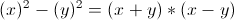. Obviously we cannot figure out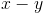since we have no information about the value of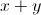.

From statement (2) only, we have no idea whatis by knowing the value of. However, putting the two statements together, we will get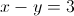.

### Example Question #2 : Solving By Factoring

Don's teacher challenged him to write two whole numbers in the square and circle in the diagram below in order to make a polynomial that could be factored.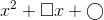Assuming that Don wrote two whole numbers, did he succeed?

Statement 1: The number Don wrote in the circle is the square of half the number he wrote in the square.

Statement 2: The sum of the numbers Don wrote in the square and in the circle is 15.

EITHER statement ALONE is sufficient to answer the question.

Statement 1 ALONE is sufficient to answer the question, but Statement 2 ALONE is NOT sufficient to answer the question.

BOTH statements TOGETHER are insufficient to answer the question.

BOTH statements TOGETHER are sufficient to answer the question, but NEITHER statement ALONE is sufficient to answer the question.

Statement 2 ALONE is sufficient to answer the question, but Statement 1 ALONE is NOT sufficient to answer the question.

Statement 1 ALONE is sufficient to answer the question, but Statement 2 ALONE is NOT sufficient to answer the question.

Explanation:

By Statement 1 alone, if we call the number in the square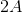, then the number in the circle is the square of half this, or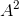; the polynomial is therefore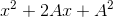,

which is the pattern of a perfect square trinomial. The polynomial can be factored, and Don succeeded.

Assume Statement 2 alone. The polynomials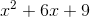and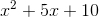fit the conditions of Statement 2.

Some trinomial of the pattern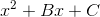can be factored as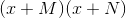, whereandhave productand sum.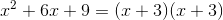, since 3 and 3 have sum 6 and product 9.

However,cannot be factored; no two integers exist with product 10 and sum 5.

### Example Question #1 : Dsq: Understanding Factoring

Karen's teacher challenged her to write two whole numbers in the square and circle in the diagram below in order to make a polynomial that could be factored.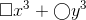Assuming both numbers are whole numbers, did Karen succeed?

Statement 1: The cube root of the number Julia wrote in the circle is a whole number.

Statement 2: The number Julia wrote in the square is twenty-seven times the number Julia wrote in the circle.

BOTH statements TOGETHER are insufficient to answer the question.

Statement 1 ALONE is sufficient to answer the question, but Statement 2 ALONE is NOT sufficient to answer the question.

Statement 2 ALONE is sufficient to answer the question, but Statement 1 ALONE is NOT sufficient to answer the question.

EITHER statement ALONE is sufficient to answer the question.

BOTH statements TOGETHER are sufficient to answer the question, but NEITHER statement ALONE is sufficient to answer the question.

Statement 2 ALONE is sufficient to answer the question, but Statement 1 ALONE is NOT sufficient to answer the question.

Explanation:

A binomial of the form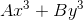can be factored out as the sum of cubes if and only if bothandare perfect cubes, and it can be factored out by taking out a GCF if the GCF ofandis not 1.

Statement 1 alone gives no clue as to the number that was placed in the square. The polynomials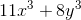and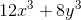fit the statement, but neither can be factored as the sum of cubes, and only the latter can have a greatest common factor (4) factored out.

Assume Statement 2 alone. There are two possible cases:

Case 1: Julia wrote a 1 in the circle.

The polynomial is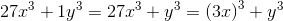, which, as the sum of cubes, is factorable.

Case 2: Julia wrote a different whole number in the circle.

Since the number in the square is 27 times the number in the circle, at the very least, the polynomial can be factored by distributing out the number in the circle. For example,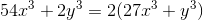### Example Question #4 : Solving By Factoring

Julia's  teacher challenged her to write two whole numbers in the square and circle in the diagram below in order to make a polynomial that could be factored.Did Julia succeed?

Statement 1: The cube root of the number Julia wrote in the circle is a whole number.

Statement 2: The number Julia wrote in the square is ten times the number Julia wrote in the circle.

Statement 2 ALONE is sufficient to answer the question, but Statement 1 ALONE is NOT sufficient to answer the question.

Statement 1 ALONE is sufficient to answer the question, but Statement 2 ALONE is NOT sufficient to answer the question.

EITHER statement ALONE is sufficient to answer the question.

BOTH statements TOGETHER are insufficient to answer the question.

BOTH statements TOGETHER are sufficient to answer the question, but NEITHER statement ALONE is sufficient to answer the question.

BOTH statements TOGETHER are insufficient to answer the question.

Explanation:

A binomial of the formcan be factored out as the sum of cubes if and only if bothandare perfect cubes, and it can be factored out by taking out a GCF if the GCF ofandis not 1.

Assume both statements to be true.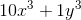- which is equal to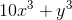- fits the conditions. But the polynomial cannot be factored using the sum of cubes property (10 is not a cube, but 1 is), nor can a greatest common factor be taken out (the greatest common factor of the terms is 1).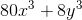fits the conditions, and can be factored out as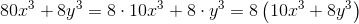### Example Question #5 : Solving By Factoring

Chad's teacher challenged him to write two whole numbers in the square and circle in the diagram below in order to make a polynomial that could be factored.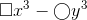Statement 1: The cube root of the number Chad wrote in the square is a whole number.

Statement 2: The cube root of the number Chad wrote in the circle is an irrational number.

BOTH statements TOGETHER are sufficient to answer the question, but NEITHER statement ALONE is sufficient to answer the question.

Statement 2 ALONE is sufficient to answer the question, but Statement 1 ALONE is NOT sufficient to answer the question.

BOTH statements TOGETHER are insufficient to answer the question.

Statement 1 ALONE is sufficient to answer the question, but Statement 2 ALONE is NOT sufficient to answer the question.

EITHER statement ALONE is sufficient to answer the question.

BOTH statements TOGETHER are insufficient to answer the question.

Explanation:

Assume both statements are true. To try to factor the polynomial, we have one of two possibilities - the difference of cubes, or a greatest common factor. But since the number in the circle is not a perfect cube, this leaves us with factoring by GCF.

The polynomial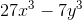fits both statements;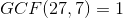, so it cannot be factored.

The polynomial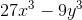fits both statements;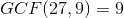, so it can be factored: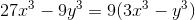### Example Question #6 : Solving By Factoring

Willy's teacher challenged him to write two whole numbers in the square and circle in the diagram below in order to make a polynomial that could be factored.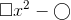Did Willy succeed?

Statement 1: The number Willy wrote in the square is a multple of 4.

Statement 2: The number Willy wrote in the circle is a multiple of 9.

BOTH statements TOGETHER are sufficient to answer the question, but NEITHER statement ALONE is sufficient to answer the question.

Statement 1 ALONE is sufficient to answer the question, but Statement 2 ALONE is NOT sufficient to answer the question.

BOTH statements TOGETHER are insufficient to answer the question.

Statement 2 ALONE is sufficient to answer the question, but Statement 1 ALONE is NOT sufficient to answer the question.

EITHER statement ALONE is sufficient to answer the question.

BOTH statements TOGETHER are insufficient to answer the question.

Explanation:

Assume both statements are true.

If Willy wrote a 4 in the circle and a 9 in the square, both statements are satisfied, and the resulting polynomial can be factored as the difference of squares: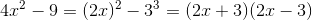If Willy wrote a 4 in the circle and a 27 in the square, both statements are satisfied. However, the resulting polynomial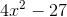is prime; no greatest common factor can be taken out, and the only possible pattern, the difference of squares, does not fit, since the square root of 27 is irrational.

The two statements together are insufficient.

### Example Question #7 : Solving By Factoring

Theresa's teacher challenged her to write whole numbers in the circle and the square in the diagram below in order to make a polynomial that could be factored.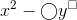Assuming Theresa wrote two whole numbers, did she succeed?

Statement 1: Theresa wrote a 64 in the circle.

Statement 2: Theresa wrote a multiple of 6 in the square.

Statement 2 ALONE is sufficient to answer the question, but Statement 1 ALONE is NOT sufficient to answer the question.

BOTH statements TOGETHER are insufficient to answer the question.

EITHER statement ALONE is sufficient to answer the question.

Statement 1 ALONE is sufficient to answer the question, but Statement 2 ALONE is NOT sufficient to answer the question.

BOTH statements TOGETHER are sufficient to answer the question, but NEITHER statement ALONE is sufficient to answer the question.

BOTH statements TOGETHER are sufficient to answer the question, but NEITHER statement ALONE is sufficient to answer the question.

Explanation:

The template fits the difference of squares pattern, which may be used only if all coefficients are perfect squares and all exponents are even (making the powers of the variables perfect squares). Each statement only answers the question of one of these qualifications; the two together answer both.

### Example Question #8 : Solving By Factoring

Consider function.

I)has zeroes atand.

II)is a second degree polynomial.

Find the equation that models.

Statement I is sufficient to answer the question, but statement II is not sufficient to answer the question.

Either statement alone is sufficient to answer the question.

Both statements are necessary to answer the question.

Statement II is sufficient to answer the question, but statement I is not sufficient to answer the question.

Explanation:

If we consider statement II, we know f(x) must be of the form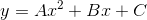.

Due to the zero product property and statement I, we know that f(x) CAN look like this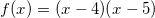.

However, it could also look like this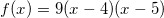.

Or this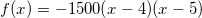.

So with what we are given it is impossible to find the true equation for f(x).

### Example Question #9 : Solving By Factoring

Simplify: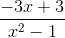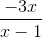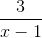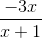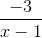Explanation:

In order to simplify, we must first pull out the largest common factor of each term in the numerator, -3: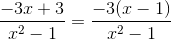We then recognize that the denominator is a difference of squares: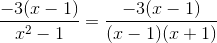We can therefore cancel the (x-1) terms and are left with: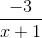Tired of practice problems?

Try live online GMAT Math prep today.